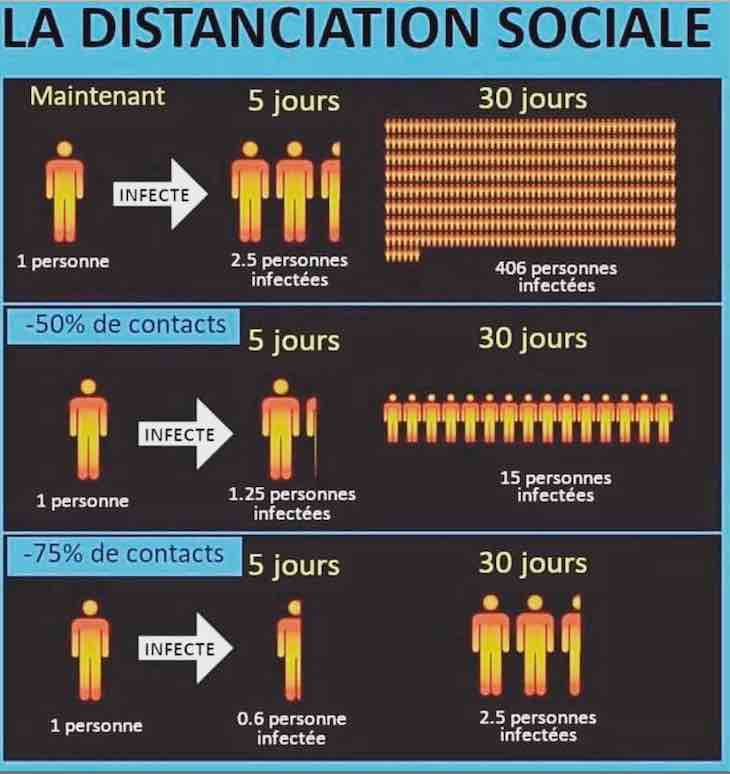WOW !! MUCH LOVE ! SO WORLD PEACE !
Fond bitcoin pour l'amélioration du site: 1memzGeKS7CB3ECNkzSn2qHwxU6NZoJ8o
Dogecoin (tips/pourboires): DCLoo9Dd4qECqpMLurdgGnaoqbftj16NvpRechercher sur le site:

# Flood vulnerability assessment of donstream area in Mono basin in Yoto district, south-eastern Togo

( Télécharger le fichier original )
par Abravi Essenam KISSI
University of Lome - Master 2014

## 3.3.3.2. Analysis of the determinants of communities' vulnerability to flood

1) Analysis of Flood Characteristics

a) Flood frequency and magnitude analysis

The magnitude of an extreme event is inversely related to its frequency of occurrence, very severe events occurring less frequently than more moderate events (Maiti, 2007, p 44). The objective of frequency of occurrence is obtained through the use of probability distributions. Some of the commonly used probability distributions are: Gumbel's or Extreme Value type 1 distribution (EV1); Log-Normal distribution; Log-Pearson type III distribution (LP3), and Method of plotting position.

For this study, further insight into flood frequency is provided by the return period analysis. The return period was obtained using the most efficient formula for computing plotting positions for unspecified distributions and now commonly used for most sample data: the Weibull equation (1). The objective of the method is to build the relation between the probability of the occurrence (return period) of a certain event and its magnitude. Frequency is how often an event of a given magnitude may be expected to occur in the log-run average.

The annual peak discharge data of the Mono River at Athieme station (1971-2010, N= 40 years) is selected for flood frequency analysis. A simple technique was to arrange the given peak in descending order of magnitude and assigned an order number (m). The probability of occurrence for each observation is given by: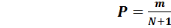(1) (Sreyasi Maiti 2007, p 45)

Where: P= Probability of occurrence; m= order number of the event; N= Total number of events in the data; The return period for each observation was determined using the following formula: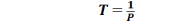(2) (Sreyasi Maiti 2007, p 45)

Where: T = return period (Recurrence interval or frequency)

Depending on the flood peaks recorded in 2010 for the study area and the average flood peaks for the examined period, floods are classified according to their magnitude.

b) Flood duration and flood water level assessment

Data on flood duration and flood water levels were obtained from each household from interview. The interviewed household could recall the peak duration of flooding during the latest more severe flood (2010). The average days recorded from household interviews was calculated for each village. Flood water levels were measured inside the house as revealed by marks on building walls with reference to the ground floor during the interviews. Only houses in the main village (populated area) were considered. The flood water levels were ranged from the lowest level to the highest level for each village.

## 3.3.3.3 Analysis of human-environmental condition

Statistical analyses were used as the methods for human-environmental condition components analysis. It includes descriptive statistics to describe all the data in general.

## 3.3.3.4 Computation of Flood Vulnerability Index (FVI)

The collected data were arranged in the form of a rectangular matrix with rows representing villages and columns representing indicators. In order to obtain figures which are free from the units and also to standardize their values, the indicators were normalized so that they all lie between 0 and 1. After computing the normalized scores the index is constructed by giving unequal weights to all indicators.

1) Normalisation of Indicators Using Functional Relationship

Two types of functional relationships are possible: vulnerability increases with increase (decrease) in the value of the indicator. The study used then two formula to normalise indicator, depending on their functional relationship with vulnerability. Then, in case that the indicator has an increase functional relationship with vulnerability (positive indicators), the normalisation is done using the following formula: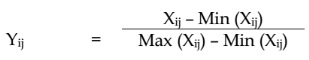(3)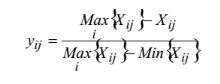On the other hand, in case that the indicator has a decrease functional relationship with vulnerability (negative indicators), the normalized score is computed using the formula:

(4)

Xij denotes the value of j indicator (j=1, 2, .........30) in the i village (i=1, 2-, ....8).

Yij is the matrix corresponding to the normalised score;

Wj and Yij lie between 0 and 1; Ó Wi = 1

It is obvious that the scaled values of Yij lies between 0 and 1. The value 1 corresponds to that village with maximum value and 0 corresponds to the village with minimum value. Through those formula the normalised scores for each indicator were obtained using MS-EXCEL Max() and Min() functions.

2) Method of Weighting and Aggregation of Indicators into Vulnerability Index

After computing the normalized scores, the index is constructed by giving an unequal weight to all indicators. In literature, several methods are used to give weight to indicators either equal weights (simple average of the scores and Patnaik and Narain Methods) or unequal weights (Expert judgement and Iyengar and Sudarshan's methods) or multivariate statistical techniques (Principal components and cluster analysis method).

The present study uses an unequal method of Iyengar and Sudarshan's to give weight to all indicators. Iyengar and Sudarshan (1982) developed a method to work out a composite index from multivariate data and it was used to rank the districts in terms of their economic performance. This methodology is statistically sound and equally suited for the development of composite index of vulnerability to climate change. In Iyengar and Sudarshan's method, the weights are assumed to vary inversely as the variance over the regions in the respective indicators of vulnerability.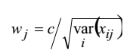That is, the weight wj is determined by:

(5)

where c is a normalizing constant: equation 6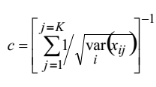(6)

The choice of the weights in this manner would ensure that large variation in any one of the indicators would not unduly dominate the contribution of the rest of the indicators and distort inter regional comparisons. It is well known that, in statistical comparisons, it is more efficient to compare two or more means after equalizing their variances.

The overall village index, Yi , also varies from zero (0) to one (1) with 1 indicating maximum vulnerability and 0 indicating no vulnerability at all. the higher the district index, the more the level of vulnerability .

The composite indicator for flood vulnerability factors (exposure, susceptibility and resilience) for the ith village was obtained as:

Yi= ?Wj Yij

(7)

where: Yi is the composite indicator of ith village; Wj is the weight for each indicator lies between 0 and 1; ?Wj= 1; and Yij is the normalised scores of indicators.

To ensure that the indices calculated for each vulnerability factor can be compared, the sum for each factor of exposure, susceptibility and resilience are divided by their respective number of indicators that describe each vulnerability factor. The composite vulnerability index for exposure factor is given as: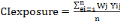(8)

Where: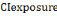is the composite vulnerability index of exposure factor,

Wj is the weight a single indicator, ei is exposure indicators; Yij is the normalised value of exposure indicator; n is the number of indicators.

Susceptibility and resilience factors can all be represented in similar way.

Any flood vulnerability analysis requires information regarding these factors, which can be specified in terms of exposure indicators, sensitivity indicators and resilience indicators. Finally, the vulnerability of a system to flood events can be expressed with the following general equation (Balica, 2007, p 37). This equation is used in the present study to compute Flood Vulnerability Index (FVI).

Vulnerability = Exposure + Susceptibility - Resilience (9)

3.3.3.5. Flood vulnerability maps

The composite index values of the three factors of vulnerability and total flood vulnerability index values were integrated in ArcGIS 10.1 software with all relevant input data being available in a digital spatial database (polygon shape file) to produce exposure, susceptibility, resilience and vulnerability maps. The maps were classified and colour coded green-yellow-red, indicating low-moderate-high areas, respectively.